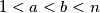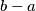### MEMO 2010 pojedinačno problem 4

Kvaliteta:
Avg: 3,0
Težina:
Avg: 4,8
Find all positive integers$n$ which satisfy the following tow conditions:
(a)$n$ has at least four different positive divisors;
(b) for any divisors$a$ and$b$ of$n$ satisfying$1, the number$b-a$ divides$n$.
Izvor: Srednjoeuropska matematička olimpijada 2010, pojedinačno natjecanje, problem 4